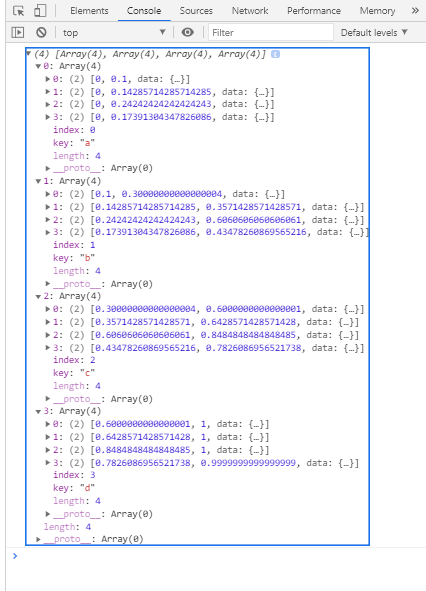# D3.js stackOffsetExpand() Method

• Last Updated : 29 Sep, 2020

The D3.js stackOrderOffsetExpand() method applies a zero baseline and normalizes the values for each point such that the topline is always one.

Syntax:

Hey geek! The constant emerging technologies in the world of web development always keeps the excitement for this subject through the roof. But before you tackle the big projects, we suggest you start by learning the basics. Kickstart your web development journey by learning JS concepts with our JavaScript Course. Now at it's lowest price ever!

```d3.stackOrderOffsetExpand(series, order);
```

Parameters: This function accepts two parameters as mentioned above and described below.

• series: This is the series based on which offset is expanded.
• order: This is the ordering of the stack.

Return Value: This method returns no value.

Example:

## HTML

 ```<``html``>``<``head``>``    ``<``meta` `charset``=``"utf-8"``>``    ``<``script` `src``=``        ``"https://d3js.org/d3.v5.min.js"``>``    `````` ` `<``body``>``    ``<``h1` `style``=``"text-align: center; color: green;"``>``        ``GeeksforGeeks``    ```` ` `    ``<``script``>``        ``var data = [``        ``{letter: {a: 1000, b: 2000, c: 3000, d: 4000}},``        ``{letter: {a: 2000, b: 3000, c: 4000, d: 5000}},``        ``{letter: {a: 640, b: 960, c: 640, d: 400}},``        ``{letter: {a: 320, b: 480, c: 640, d: 400}}``        ``];``        ``var stack = d3.stack()``            ``.keys(["a", "b", "c", "d"])``            ``.value((d, key) => d.letter[key])``            ``.order(d3.stackOrderNone)`` ` `            ``// Use of d3.stackOrderOffsetExpand() Method``            ``.offset(d3.stackOffsetExpand);`` ` `        ``var series = stack(data);``        ``console.log(series);``    ```````

Output:My Personal Notes arrow_drop_up